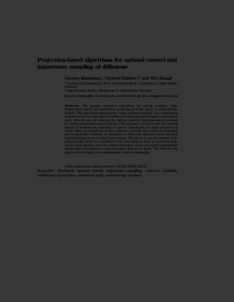Repository: Freie Universität Berlin, Math Department

# Model reduction algorithms for optimal control and importance sampling of diffusions

Hartmann, C. and Schütte, Ch. and Zhang, W. (2016) Model reduction algorithms for optimal control and importance sampling of diffusions. Nonlinearity, 29 (8). pp. 2298-2326. ISSN 0951-7715Preview
PDF - Submitted Version
866kB

## Abstract

We propose numerical algorithms for solving complex, high- dimensional control and importance sampling problems based on reduced-order models. The algorithms approach the “curse of dimensionality” by a combination of model reduction techniques for multiscale diffusions and stochastic optimization tools, with the aim of reducing the original, possibly high-dimensional problem to a lower dimensional representation of the dynamics, in which only few relevant degrees of freedom are controlled or biased. Specifically, we study situations in which either an suitable set of slow collective variables onto which the dynamics can be projected is known, or situations in which the dynamics shows strongly localized behaviour in the small noise regime. The idea is to use the solution of the reduced-order model as a predictor of the exact solution that, in a corrector step, can be used together with the original dynamics, where no explicit assumptions about small parameters or scale separation have to be made. We illustrate the approach with simple, but paradigmatic numerical examples.

Item Type: Article Mathematical and Computer Sciences > Mathematics > Applied Mathematics Department of Mathematics and Computer Science > Institute of MathematicsDepartment of Mathematics and Computer Science > Institute of Mathematics > Cellular Mechanics GroupDepartment of Mathematics and Computer Science > Institute of Mathematics > BioComputing Group 1552 Carsten Hartmann 22 Jun 2015 12:19 03 Mar 2017 14:41

Repository Staff Only: item control page